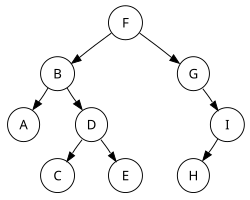## Wednesday, November 19, 2008

### ExampleIn this binary search tree, Preorder traversal sequence: F, B, A, D, C, E, G, I, HInorder traversal sequence: A, B, C, D, E, F, G, H, I Note that the inorder traversal of this binary search tree yields an ordered list Postorder traversal sequence: A, C, E, D, B, H, I, G, FLevel-order traversal sequence: F, B, G, A, D, I, C, E, H

### Sample implementations

`preorder(node) print node.value if node.left ≠ null then preorder(node.left) if node.right ≠ null then preorder(node.right)`
`inorder(node) if node.left  ≠ null then inorder(node.left) print node.value if node.right ≠ null then inorder(node.right)`
`postorder(node) if node.left  ≠ null then postorder(node.left) if node.right ≠ null then postorder(node.right) print node.value`

All sample implementations will require stack space proportional to the height of the tree. In a poorly balanced tree, this can be quite considerable.

We can remove the stack requirement by maintaining parent pointers in each node, or by threading the tree. In the case of using threads, this will allow for greatly improved inorder traversal, although retrieving the parent node required for preorder and postorder traversal will be slower than a simple stack based algorithm.

### Testing Challenge

Can you find more than 20 defects in the below image? Write your defects in the comments section.